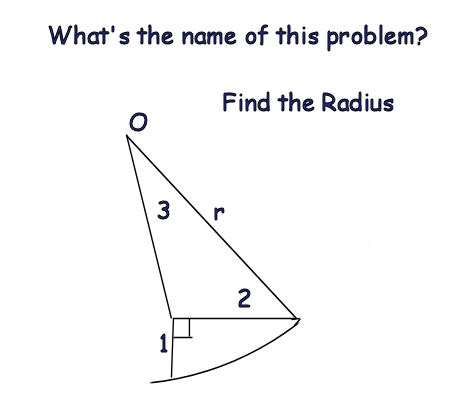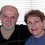# Can You Help ?I usually save the interesting ones in a set for future examination but I didn't this time and I think I'm on the way to solving it.

This is the setup:Note by Guiseppi Butel
6 years, 8 months ago

This discussion board is a place to discuss our Daily Challenges and the math and science related to those challenges. Explanations are more than just a solution — they should explain the steps and thinking strategies that you used to obtain the solution. Comments should further the discussion of math and science.

When posting on Brilliant:

• Use the emojis to react to an explanation, whether you're congratulating a job well done , or just really confused .
• Ask specific questions about the challenge or the steps in somebody's explanation. Well-posed questions can add a lot to the discussion, but posting "I don't understand!" doesn't help anyone.
• Try to contribute something new to the discussion, whether it is an extension, generalization or other idea related to the challenge.

MarkdownAppears as
*italics* or _italics_ italics
**bold** or __bold__ bold
- bulleted- list
• bulleted
• list
1. numbered2. list
1. numbered
2. list
Note: you must add a full line of space before and after lists for them to show up correctly
paragraph 1paragraph 2

paragraph 1

paragraph 2

[example link](https://brilliant.org)example link
> This is a quote
This is a quote
    # I indented these lines
# 4 spaces, and now they show
# up as a code block.

print "hello world"
# I indented these lines
# 4 spaces, and now they show
# up as a code block.

print "hello world"
MathAppears as
Remember to wrap math in $$ ... $$ or $ ... $ to ensure proper formatting.
2 \times 3 $2 \times 3$
2^{34} $2^{34}$
a_{i-1} $a_{i-1}$
\frac{2}{3} $\frac{2}{3}$
\sqrt{2} $\sqrt{2}$
\sum_{i=1}^3 $\sum_{i=1}^3$
\sin \theta $\sin \theta$
\boxed{123} $\boxed{123}$

Sort by:

I'm getting a value of $r = \displaystyle\sqrt{\dfrac{53 + 6\sqrt{19}}{5}}$,

which equals $3.9788$ rounded to $4$ decimal places. Is that what you got? I'll write up my method shortly.

Edit: Label the triangle so that $OA = 3$ and $AB = 2$. Also, label the length $1$ line segment $AP$ and let $\angle OAB = \theta$.

Then $OP = r$ and $\angle OAP = \frac{3\pi}{2} - \theta$. We can now apply the Cosine Law to triangles $\Delta OAB$ and $\Delta OAP$ to obtain the equations

(i) $r^{2} = 3^{2} + 2^{2} - 2*3*2*\cos(\theta) \Longrightarrow 13 - 12\cos(\theta)$, and

(ii) $r^{2} = 3^{2} + 1^{2} - 2*3*1*\cos(\frac{3\pi}{2} - \theta) \Longrightarrow 10 + 6\sin(\theta)$.

Equating (i) and (ii) we then have that

$13 - 12\cos(\theta) = 10 + 6\sin(\theta) \Longrightarrow 1 - 4\cos(\theta) = 2\sin(\theta)$.

Now square both sides and simplify to find that

$1 - 8\cos(\theta) + 16\cos^{2}(\theta) = 4*(1 - \cos^{2}(\theta)) \Longrightarrow 20\cos^{2}(\theta) - 8\cos(\theta) - 3 = 0$,

which has solutions $\cos(\theta) = \dfrac{8 \pm \sqrt{64 + 4*3*20}}{2*20} = \dfrac{1}{5} \pm \dfrac{\sqrt{19}}{10}$.

Now from the diagram we are looking for $\theta \gt \frac{\pi}{2}$, i.e., $\cos(\theta) \lt 0$, se we take the root

$\cos(\theta) = \dfrac{1}{5} - \dfrac{\sqrt{19}}{10}$.

Now substitute this value into (i) to find that

$r^{2} = 13 - 12*(\dfrac{1}{5} - \dfrac{\sqrt{19}}{10}) = \dfrac{53 + 6\sqrt{19}}{5}$,

and so $r = \displaystyle\sqrt{\dfrac{53 + 6\sqrt{19}}{5}}$.

- 6 years, 8 months ago

Hi, Brian, what's the name of the problem?

- 6 years, 8 months ago

Oh, sorry, you were just wanting a name. I have no idea if it has a specific name, but it seems to be an exercise in the application of the Cosine Law. I can think of one other way of trying to solve it, but I haven't worked out the details of that approach yet.

I'm sorry if I spoiled this question for posting. I just saw it as an interesting problem and immediately had to solve it. You could delete this note and then post the problem after; I would be fine with that. :)

- 6 years, 8 months ago

- 6 years, 7 months ago

Yeah, I know. I don't think it's a big problem, since this posts has no likes or re-shares. Sorry about this; I had no idea about the question's "history" at the time I posted my solution. :(

- 6 years, 7 months ago

Nah, it's fine. You also forgot to prove that $\theta > \dfrac{\pi}{2}$, although it may be obvious.

- 6 years, 7 months ago

O.k., I'm glad. And yes, I did gloss over that detail, and even though it does seem obvious it would require a proof. At this stage, though, I'm just going to stick with "And clearly, $\theta \gt \dfrac{\pi}{2}$". :)

- 6 years, 7 months ago

I think I did see this problem somewhere else posted in Brilliant, but I can't think of the name of either the problem or the creator. Brian's answer is correct, though.

Okay, I've found the problem, posted by [Deleted]

[Deleted]

- 6 years, 8 months ago

Well, that made it easy for me to solve [deleted]'s question, then. :) Having found a solution assuming that $\theta$ was obtuse it is clear that $\theta$ can be obtuse, but I neglected to prove that $\theta$ would necessarily have to be obtuse. I see that in response to [deleted]'s solution to his own problem, Ariel Gershon has proved this to be the case.

- 6 years, 8 months ago

lol free rating...

- 6 years, 8 months ago

Yeah, I know. I wonder if I should delete my answer now. I had no idea the question had been asked on Brilliant before until Michael posted the link. It's not fair to [deleted] if people can now cheat on his question, but Guiseppi asked this question independently so I don't want to delete my answer to his question.

- 6 years, 8 months ago

i dont know.... , i think u should delete it.

- 6 years, 8 months ago

Problem solved. No more free points. :)

- 6 years, 8 months ago

lol

no free shot to lv5 geometry attempt

- 6 years, 8 months ago

without it, i will never solve it

- 6 years, 8 months ago

Hi Michael. In light of Math Man's comment below, I'm wondering if it's a good idea having the link to [deleted]'s question posted here. whatever you decide is fine, but I just thought I should give you a heads up. I could delete my answer, but then that would defeat the point of Guiseppi's post.

- 6 years, 8 months ago

Okay, deleted, as I noticed that problem has just now been reshared.

- 6 years, 8 months ago

Thanks, Michael. I've inserted a few [deleted]'s as well.

- 6 years, 8 months ago

its title is this: [deleted]

Moderator's note: please do not post spoilers

- 6 years, 6 months ago